# White Paper – Analysis of Input Characteristic for a UPS Connected to a Generator

### Our History of Power Product##### 1. Introduction

In the age of information technology, application of the uninterruptable power supply (hereafter, “UPS”) is widespread to meet the requirement of stable supply power for critical loads such as computers. Also, a generator can be used as the input for the UPS when emergency power is required for long periods of commercial power failure. When a generator is connected to a UPS, sometimes, the problem of Sub Synchronous Resonance (hereafter, “SSR”) can arise. The generator consists of a rotor, an external driving engine, and a shaft which connect the rotor with the external driving engine. Generally, the inertias of the rotor and that of the external driving engine are different. Therefore, when sudden changes in load occur resulting in a disturbance, SSR is generated due to the rotational speed difference between the generator and the rotor. In some cases, the resulting oscillation is so severe as to break the shaft between generator and rotor. It is one example of SSR. Also, in situations where the disturbance occurs, output voltage and frequency of the generator varies. Under these conditions, the UPS is controlled as constant power control and phase control and so on. So, the SSR is affected by the inertias of the rotor and external driving engine in addition to a close relationship with the UPS control (1)-(2). The input characteristic on the UPS side is analyzed in this paper. The following points are described to clarify the relationship with SSR.

1) The control method of UPS (rectifier)

2) UPS input characteristic analysis using Bode diagrams

3) Verification by waveform simulation and experiments

##### 2. Control Method of UPS (Rectifier)Fig.1 Model of UPS

Fig.1 shows the basic configuration when a generator is connected to the UPS. There are many possible UPS types, but in this example, we would like to take the widely used double conversion type UPS that consists of the voltage type rectifier and inverter. In the double conversion type UPS, a generator, a rectifier, an inverter, and a load are connected sequentially. The control system of the inverter achieves an output with constant frequency and constant voltage that is matched to the commercial power. In other words, if the load is constant, a continuous power will be supplied.

Moreover, the control system of this inverter allows a minimal change in the output voltage even when there is a sudden change in load due to the adoption of an instantaneous voltage control system. Therefore, the inverter outputs power corresponding to the load immediately after the load changes. Also, because the rectifier control system is controlling the direct current of the rectifier to keep voltage constant and because there is a relatively big electrolytic capacitor, the direct current voltage is approximately constant as well. Therefore, the inverter can be recognized as a constant current source as seen by the rectifier. Thus, in the model, we assume the inverter part as a resistive load R and input current iRL as a constant.

Now, let’s take a look at the control system of the rectifier. In addition to the function mentioned above, that maintains constant DC voltage, the rectifier control system is used to change the input current into a sine wave. As said before, when the load suddenly varies, there is a voltage and frequency fluctuation at the generator output. However, as to the voltage fluctuation, the rectifier input current changes in proportion to the rectifier input voltage (generator output voltage) since the rectifier maintains constant power. If this response is delayed, a pulsation will be generated in power between the generator and the rectifier. The same principle applies to the frequency fluctuation. When PLL (Phase Locked Loop) is used for the rectifier control system, a phase difference will be generated between the generator output and synchronous signal of the rectifier if the PLL cannot follow the frequency fluctuation. It will result in a power pulsation between the generator and the rectifier. The rectifier with a phase control method will behave in the same way (3).

With that consideration, it is necessary to clarify the rectifier control system when there is a generator output fluctuation (voltage and frequency). In the case of Sanyo’s UPS, if there is a frequency fluctuation, there will be no power pulsation thanks to the PWM control method for the rectifier that doesn’t use PLL technology. The control characteristics during a voltage fluctuation are going to be explained to simplify the analysis. Let’s begin with an explanation of the control method for the rectifier. Fig.2 shows the control block diagram for the direct current voltage, Edc (direct current voltage command value is Edc*). DC voltage, Edc, is detected and filtered by a first order filter (the delay element: Td). The filtered signal is compared to the command value resulting in an error voltage. The error voltage is processed using the PID controller (the proportional element: kd, integral element: T1, and differential element: T2), which creates the input current command value. The input current command value is compared with the input current. The resulting error signal goes through a proportional controller (proportional gain ki), and PWM control is then used to control the input current and the DC output voltage. By looking carefully at the variable component Δic of the current ic which flows in the capacitor, the relationship between the variable components of the input voltage Δ Ei, of the control signal Δec, and the capacitor current Δic can be shown as below.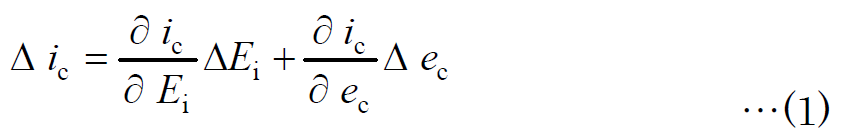ΔEi: Variable component of input voltage [V]

Δec: Variable component of control signal (input current command value) [A]

Capacitor current ic results from PWM control in the rectifier and can be put in the expression as shown below.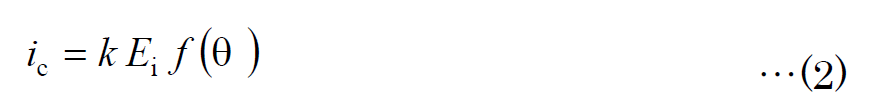Where f (θ) is a function of the angle θ as in the PWM control, if capacitor current ic changes linearly within the range of the control signal fluctuation Δec, then we can define the expression as shown below.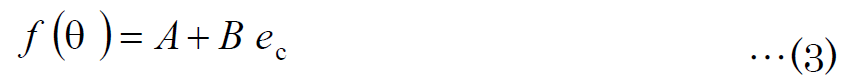Therefore, expression (2) can be rewritten as follows.Additionally, if a change of Δic is generated in the capacitor current, it can be put in the expression as shown below.The fluctuation component of the capacitor current Δic can then be expressed as follows from expressions (4) and (5).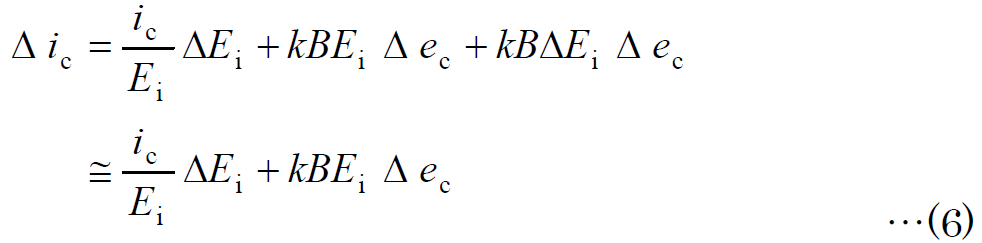The third term on the right side in the above expression was disregarded because it is much smaller than term 1 and term 2. As a result, expression (1) can be rewritten as shown by the final result in expression (6). Because the relation in expression (6) holds in the PWM control part of Fig.2, the block diagram of the variable component Δiac of the input current to the variable element ΔEi of the input voltage is as shown in Fig.3. This figure is derived from Fig.2 while assuming the following conditions.

1) The inverter part is considered as a fixed load since it is regarded as a current source, and the rectifier load current is obtained by adding resistance R to the DC capacitor.

2) Current ic which flows into the DC capacitor C was set to √3 times the input current iac of each phase because three-phase power needs to be taken into consideration (the input current of each phase goes through the three-phase full-wave rectification and flows into the DC capacitor).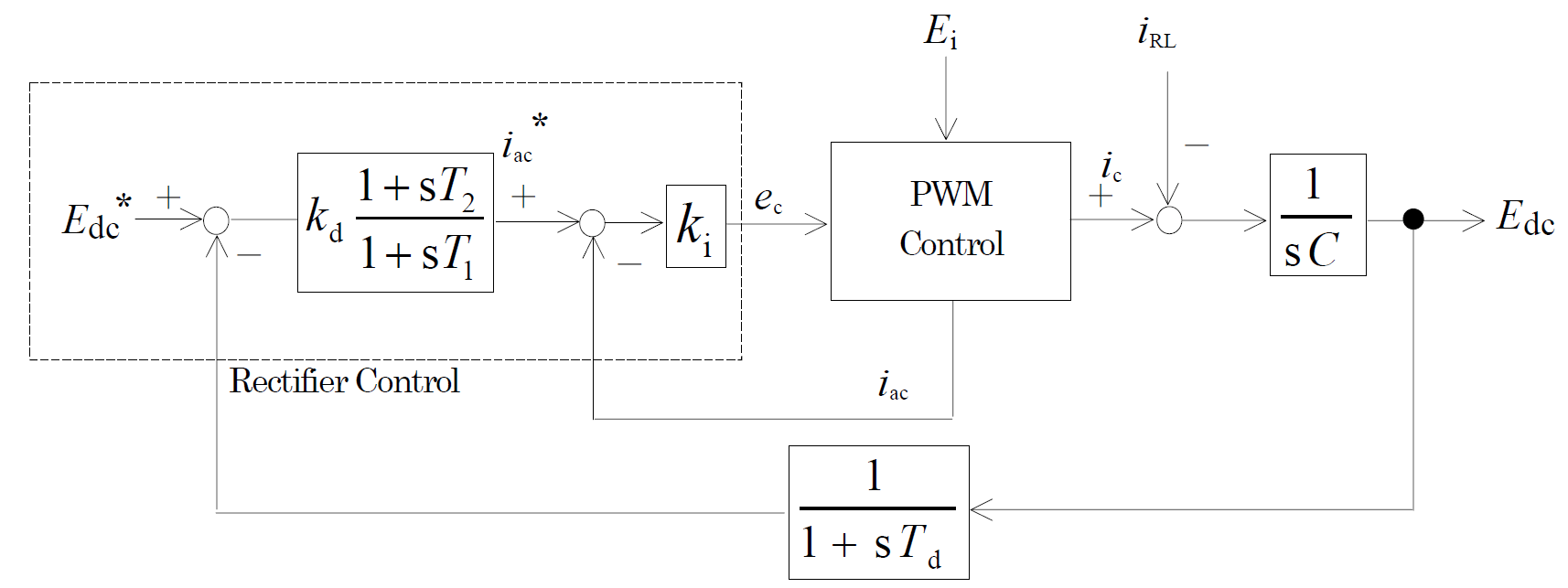3) Consideration of three-phase power, again, the conversion factor k1 of the following expression is used when creating the input current command value per phase from the error signal of DC voltage.where P is the capacity of UPS [W]

4) The input current is assumed to be following the input current command value as determined by the rectifier control disregarding any actual error due to the dead time, etc. In other words, the input current command value and the input current are assumed to be equal.

5) The line current indicates the input current. Since the input current command value created by the controller is the phase sequence current, it is converted to line current using the factor of √3

Therefore, the transfer function for the variable component of the input current to that of the input voltage can be derived from Fig.3 and is shown in the next expression.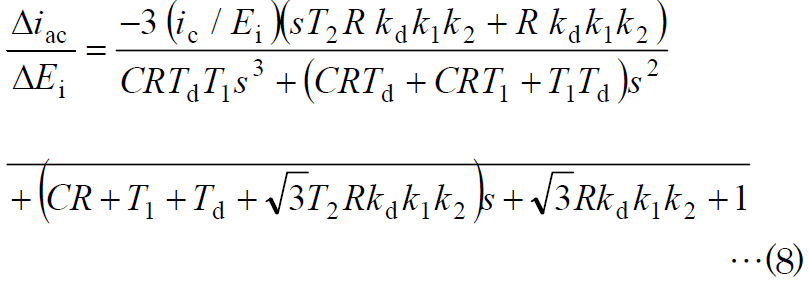where k2 is the expression shown below.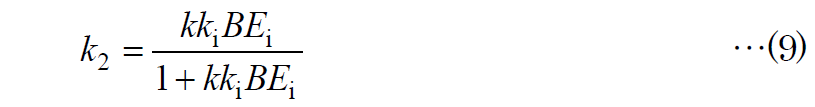######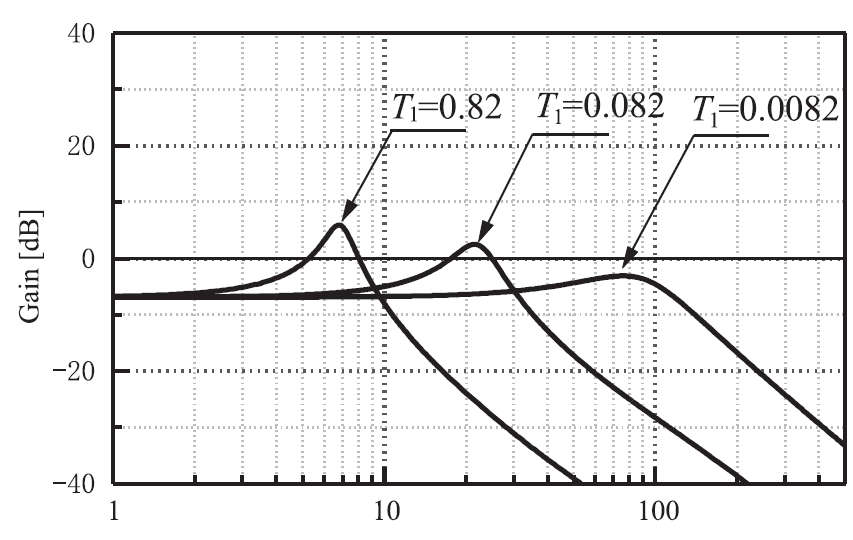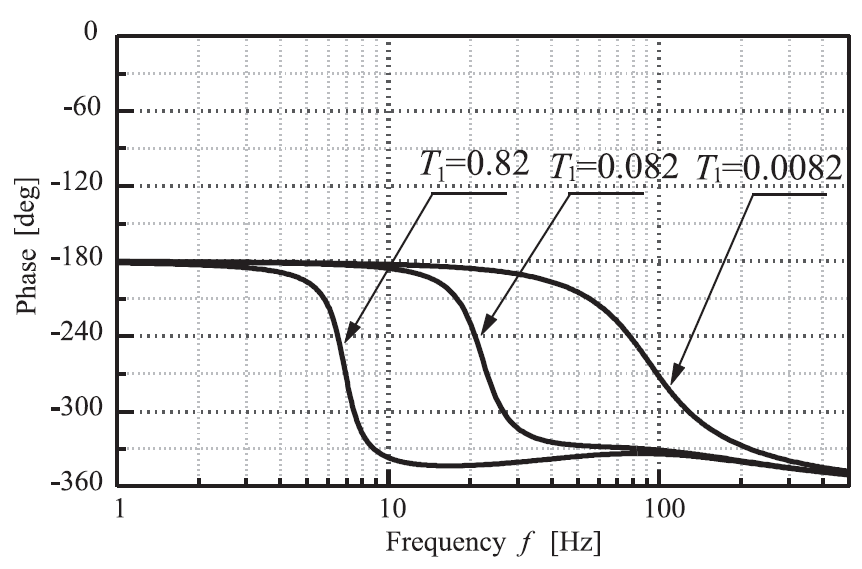Fig.4 Frequency characteristics when the integration element T1 of PID control is changed

##### 3. UPS Input Characteristic Analysis by Bode Diagrams

The characteristics of our double conversion type UPS (20kVA) are analyzed by the Bode diagram using the expression (8). Fig.4 is the frequency characteristic curves when the integral element T1 in the PID control is assumed as a parameter. Fig.5 is the frequency characteristic curves when plotting differential element T2 as a parameter. Fig.6 is the case when considering the delay element Td of the DC voltage detection as a parameter. Also, the main circuit and control values are shown in Table 1. In Fig.4, larger integral element T1 results in higher peak values and Q tends to become higher. In Fig.5, when differential element T2 is large, the peak values are reduced with a damping effect. Therefore, Q rises when T2 becomes a smaller value. Higher Q also results from an increasing delay element Td in the DC voltage detection as shown in Fig.6. In other words, changes in the integral element T1 can change the resonance frequency and increasing the differential element T2 can control the peak of the resonance. Moreover, the delay element Td is also an essential element in the behavior of the system.Suppose there is no delay element and the control is only P control (T1=0, T2=0, Td=0) then expression (8) becomes a first-order delay system and vibration is not generated. In other words, we can say that the PID control and the delay element are the causes of the resonance phenomenon. However, it is not practical to have only P control because it is understood that regular deflection would increase.

Moreover, the DC voltage control demands high accuracy because the storage battery is charged by the DC voltage control of the rectifier. It is another reason that the PID control for regular deflection is generally used. In short, the PID control should be used for the DC voltage control system, and sufficient attention needs to be paid in setting the PID parameters around the low-frequency area.##### 4. Verification by Waveform Simulation and Experiment

Waveforms were calculated from a simulation (using the Euler method when the modulation frequency was 15Hz and the amplitude ratio of the modulation frequency to a fundamental wave was 10%), and they are compared with actual measurement values as shown in Fig.7. The modulation element of the input current is 180 [deg] behind that of the input voltage because the rectifier has a constant power characteristic. The simulation waveform has a reasonable correlation to the measurement values. The simulated waveform in the frequency domain (using our double conversion type UPS (20 kVA)), the measurement value, and the value obtained from the expression (8) were compared and shown in Fig.8. Amplitude aA of the modulation element can be found by FFT (Fast Fourier Transform) since modulation signal Ex can be displayed as in the next expression.In Fig.8, the value obtained from the waveform simulation and the measurement value is a very close match at lower frequencies as compared with the value obtained from expression (8). An actual rectifier has a LC filter connected in the input to remove the high harmonics generated by the PWM control. It seems that the error appeared in the high harmonic area because this LC filter was not considered in the block diagram of Fig.3. Thus, the corresponding results for the input current characteristic to a change of input voltage were obtained between the calculation value, the simulation value, and the measurement value in the low-frequency area. It shows that a frequency can which the input current resonates when the input voltage changes and SSR are generated when this resonance frequency corresponds to the axis resonance frequency of the generator. By the way, an example of the diagram of the rotation speed to the torque of the generator is shown in Fig.9. As described above, the axis resonance frequency on the generator side exists around the resonance point of the UPS input characteristic.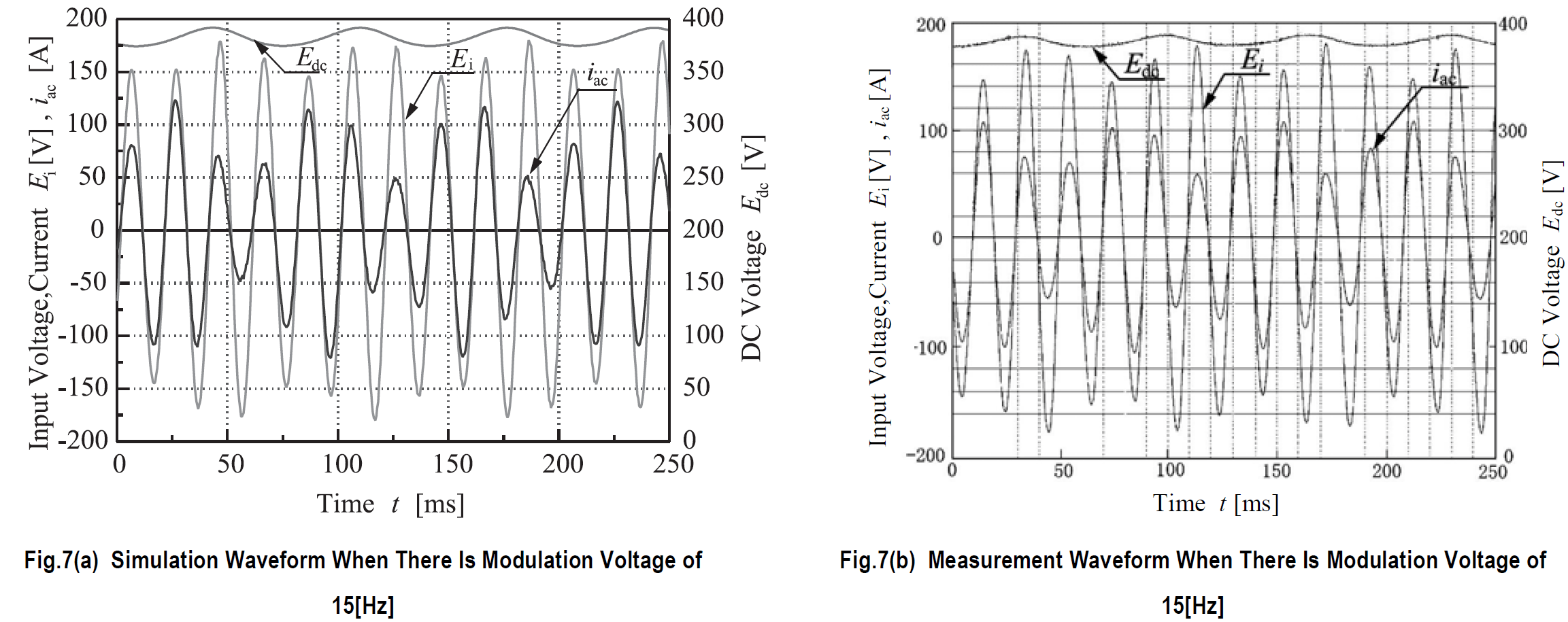The transfer function for the system of the entire generator is too complex to practically model, but to simplify, suppose the rotation speed is proportional to the generator output voltage and the torque is proportional to the generator output current, it is then possible to say that Fig.9 on top of Fig.8 is a transfer function of the system. Therefore, the stability of the system can be determined from both figures, and there is a possibility that SSR is generated when both resonance points are corresponding.

##### 5. Conclusion

In this paper, the UPS input characteristic considering a change in the input voltage was analyzed. The following points were described.

1) The transfer function for the variable component of the input current to that of the input voltage was shown, and the frequency characteristics were compared with simulation and measurement values.

2) It was revealed that the compared values almost correspond and that a resonance frequency exists in the above-mentioned frequency characteristics. Therefore, there is a possibility that SSR will be generated when the resonance frequency is similar to the axis resonance frequency of the generator.

3) It was suggested to pay particular attention to the frequency characteristic in the low frequency area when designing the control system for a rectifier. We will examine detailed stability of the total system including the generator in the future.

Reference

1) S. Roy, et al., “Stability Considerations for a Single Phase Generator – Rectifier Interface”, Intelec1998, pp.151-155.

2) Yoshiaki Okui：“A Consideration Concerning The Interface of A Generator and UPS”, 2002 Lecture Thesis Collection of The Inst. of Electrical Engineers National Convention, No.4-002, pp.3-4 (2002).

3) Hidehiro Hanazato, Tetsu Fukiage：“Stability of The Combination System of An Emergency Generator and UPS”, 2002 Lecture Thesis Collection of The Inst. of Electrical Engineers National Convention, No.121, pp.508-509 (1992). Yoshiaki Okui Joined the company in 1992 Power Systems Division, 1st Design Dept. Worked on the design and development of the UPS A doctor of engineering